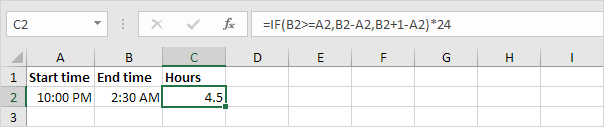Use the TIME function in Excel to add or subtract hours, minutes and seconds. To add up times in Excel, simply use the SUM function.

### Add Hours, Minutes and Seconds

Remember, to enter a time, use the ":" (colon).

1. Enter a time into cell A1.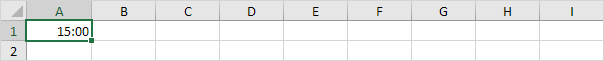2. To add 2 hours and 30 minutes to this time, enter the formula shown below.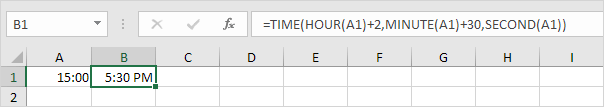Explanation: the TIME function has 3 arguments. First, use HOUR, MINUTE and SECOND to create the time entered in cell A1. Next, add 2 hours and 30 minutes.

To change the Time format, execute the following steps.

3. Select cell B1.

4. Right click, and then click Format Cells (or press CTRL + 1).

5. In the Category list, select Time, and select a Time format.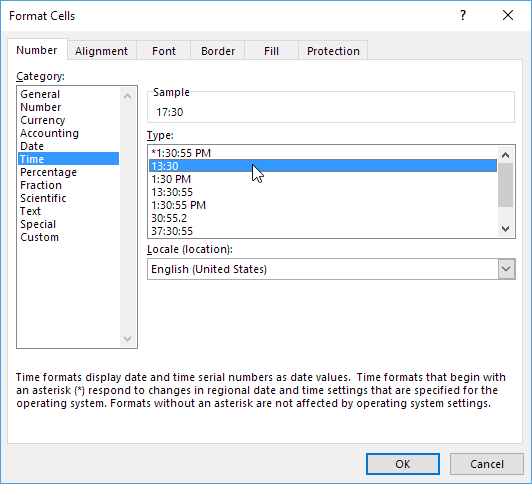6. Click OK.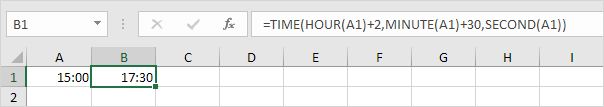7. Enter a time into cell A1 (use 2 colons to include seconds).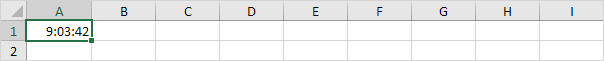8. To add 10 seconds to this time, enter the formula shown below.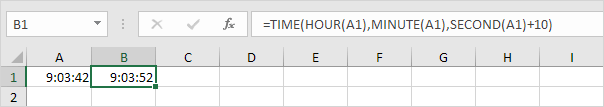Note: we changed the Time format to 13:30:55 (see step 5).

To add up times in Excel, simply use the SUM function. To display a total of more than 24 hours, change the Time format.

1. For example, task A requires 8 hours to complete, task B requires 4 hours and 30 minutes to complete, etc.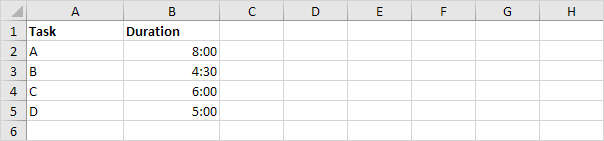2. To calculate the total duration, simply use the SUM function.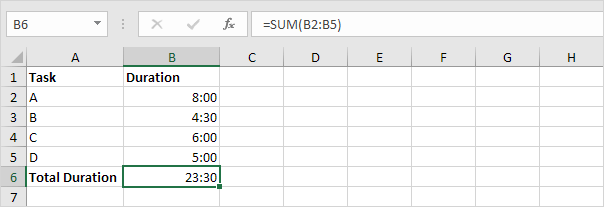3. Change the duration of task D from 5 hours to 7 hours.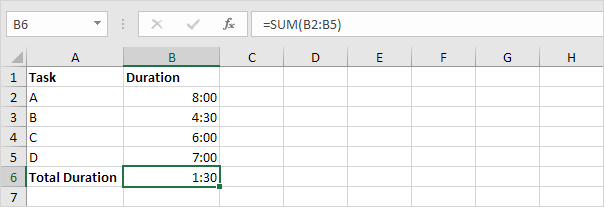To display a total of more than 24 hours, execute the following steps.

4. Select cell B6.

5. Right click, and then click Format Cells (or press CTRL + 1).

6. Select the Custom category.

7. Type the following number format code: [h]:mm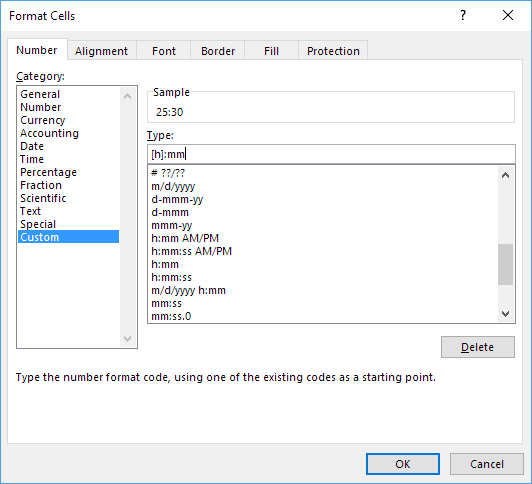8. Click OK.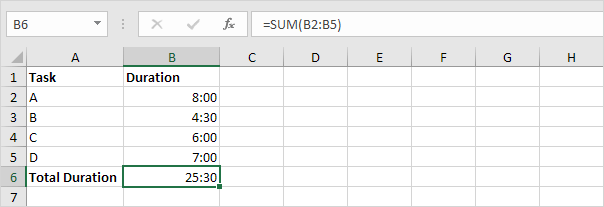### Subtract Minutes

To subtract hours, minutes or seconds from a time, use the TIME function (and HOUR, MINUTE and SECOND).

1. Enter a time into cell A1.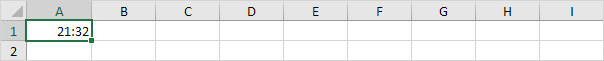2. To subtract 33 minutes from this time, enter the formula shown below.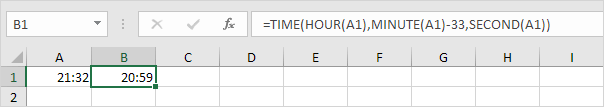### Time Difference

Calculating the difference between two times in Excel can be tricky.

1. To calculate the difference between two times in the same day, simply subtract the start time from the end time.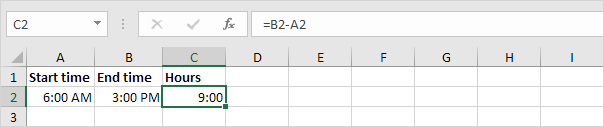Note: we changed the Time format to 13:30 (see step 5 under "Add Hours, Minutes and Seconds").

2. This simple formula doesn't work if the start time is before midnight and the end time is after midnight. The Time Difference formula below always works.3. The formula below calculates the difference between two times in hours as a decimal value.